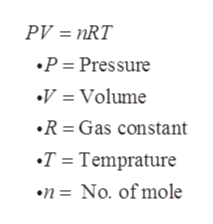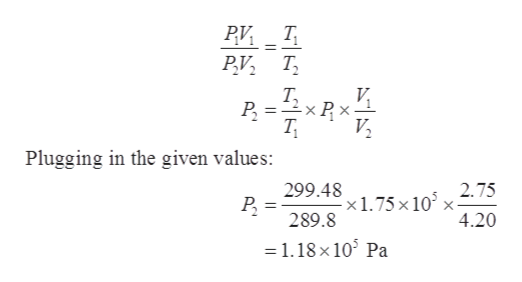# A fixed amount of a particular ideal gas at 168C and a pressure of 1.75 3 105 Pa occupies a volume of 2.75 m3. If the volume is increased to 4.20 m3 and the temperature is raised to 26.48C, what will be the new pressure of the gas?

Question
8 views

A fixed amount of a particular ideal gas at 168C and a pressure of 1.75 3 105 Pa occupies a volume of 2.75 m3. If the volume is increased to 4.20 m3 and the temperature is raised to 26.48C, what will be the new pressure of the gas?

check_circle

Step 1

Given values:

Initial pressure, P1 = 1.75 x 105 Pa

Final pressure, P2 =?

T1 = 16.8 oC = 16.8 + 273 = 289.8 K

T2 = 26.48 oC = 299.48 K

V1 = 2.75 m3

V2 = 4.20 m3

Step 2

The ideal gas equation:help_outlineImage TranscriptionclosePV nRT .P = Pressure V = Volume R=Gas constant T = Temprature n= No. of mole fullscreen
Step 3

From the ideal gas...help_outlineImage TranscriptionclosePV T V Р. =2xP Plugging in the given values: 299.48 2.75 - x 1.75 x 10° x- 4.20 289.8 1.18 x 10 Pa fullscreen

### Want to see the full answer?

See Solution

#### Want to see this answer and more?

Solutions are written by subject experts who are available 24/7. Questions are typically answered within 1 hour.*

See Solution
*Response times may vary by subject and question.
Tagged in

### Other